## Bracket Polynomial

A one-variable Knot Polynomial related to the Jones Polynomial. The bracket polynomial, however, is not a topological invariant, since it is changed by type I Reidemeister Moves. However, the Span of the bracket polynomial is a knot invariant. The bracket polynomial is occasionally given the grandiose name Regular Isotopy Invariant. It is defined by(1)

whereandare the splitting variables,''runs through all states'' ofobtained by Splitting the Link,is the product of splitting labels'' corresponding to, and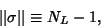(2)

where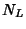is the number of loops in. Letting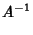(3)(4)

gives a Knot Polynomial which is invariant under Regular Isotopy, and normalizing gives the Kauffman Polynomial X which is invariant under Ambient Isotopy. The bracket Polynomial of the Unknot is 1. The bracket Polynomial of the Mirror Imageis the same as for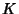but withreplaced by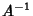. In terms of the one-variable Kauffman Polynomial X, the two-variable Kauffman Polynomial F and the Jones Polynomial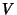,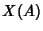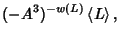(5)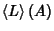(6)(7)

whereis the Writhe of.

References

Adams, C. C. The Knot Book: An Elementary Introduction to the Mathematical Theory of Knots. New York: W. H. Freeman, pp. 148-155, 1994.

Kauffman, L. New Invariants in the Theory of Knots.'' Amer. Math. Monthly 95, 195-242, 1988.

Kauffman, L. Knots and Physics. Teaneck, NJ: World Scientific, pp. 26-29, 1991.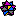Weisstein, E. W. Knots and Links.'' Mathematica notebook Knots.m.